## 螢幕截圖

•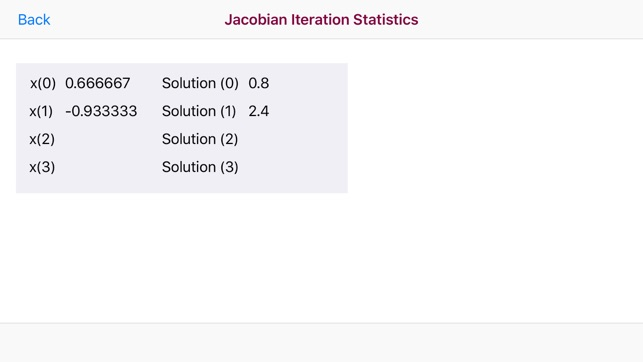•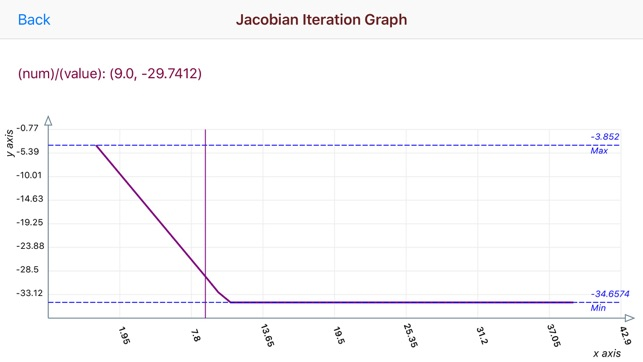•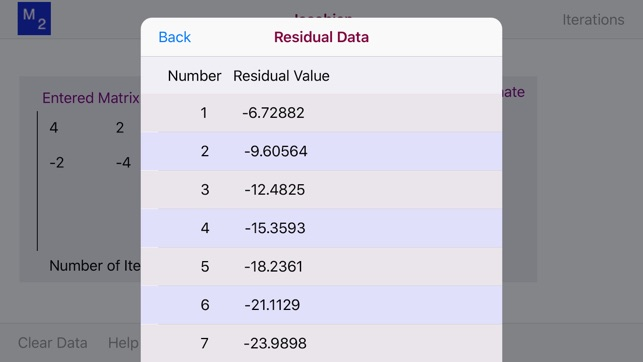•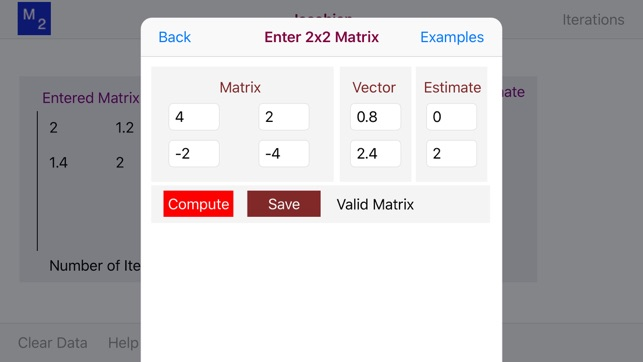•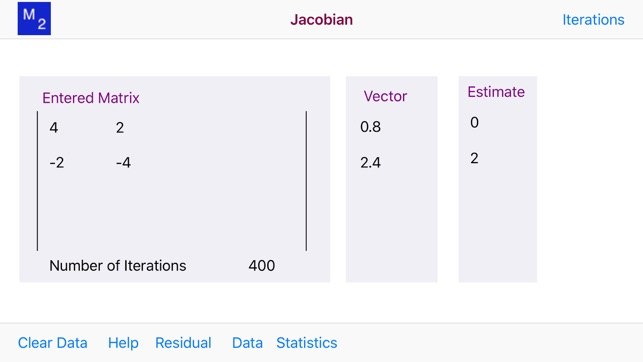## 簡介

In linear algebra, the Jacobi iterative method is an algorithm for determining the solutions of a diagonally dominant system of linear equations. Each diagonal element is solved for, and an approximate value is plugged in.

The Jacobi method is a method of solving a matrix equation on a matrix that has no zeros along its main diagonal Each diagonal element is solved for, and an approximate value plugged in. The process is then iterated until it converges.

The Jacobi Iteration Calculator IPad app allow for the easy entry of either 2x2, 3x3 or 4x4 matrices, the vector and estimate and validates the entered matrix, vector and estimate for the validity as a Jacobi matrix for iteration calculation.

The Jacobi Iteration Calculator IPhone app allow for the easy entry of either a 2x2, matrix, the vector and estimate and validates the entered matrix, vector and estimate for the validity as a Jacobi matrix for iteration calculation.

The app then determines the solution of a linear equation by the Gauss-Jacobi method. This app also calculates and graphs residual values.

## 新內容

Upgrade to iOS 12

## 資料

Donald Schaefer

7.8 MB

Copyright
© 2014 Donald Schaefer

## 支援

•